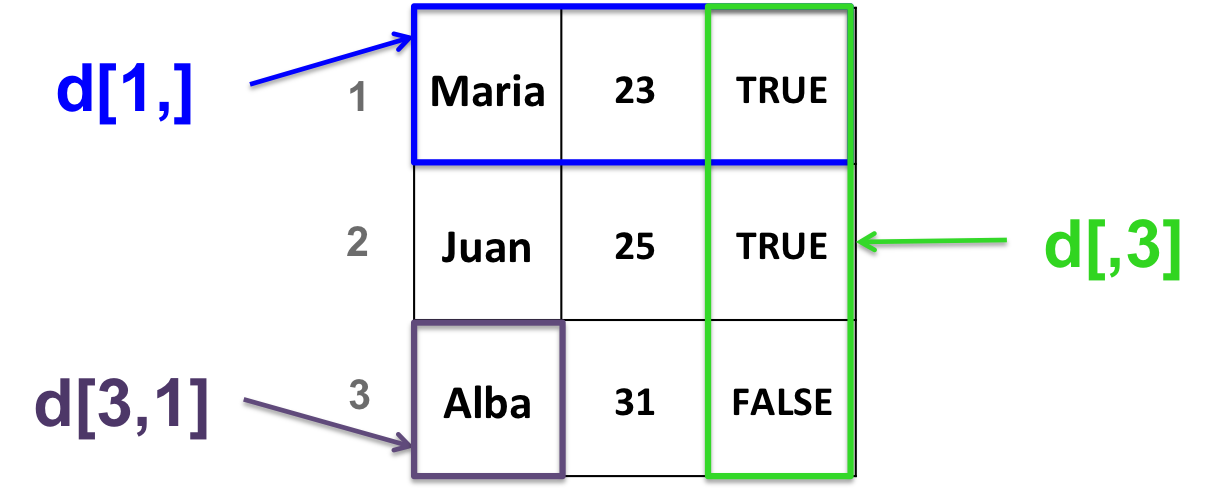## 9.6 Data frames

A data frame is a 2-dimensional structure.
It is more general than a matrix.

All columns in a data frame:

• can be of different types (numeric, character or logical)
• must have the same length

A data frame is organized by column: columns are variables and rows are observations of each variable.

### 9.6.1 Create a data frame

• With the data.frame function:
``````d <- data.frame(c("Maria", "Juan", "Alba"),
c(23, 25, 31),
c(TRUE, TRUE, FALSE))``````

NOTE: if you are working with a version of R < 4.0, you need to set the “stringsAsFactors” argument to FALSE ! Here is why:

``````# stringsAsFactors: ensures that characters are treated as characters and not as factors
d <- data.frame(c("Maria", "Juan", "Alba"),
c(23, 25, 31),
c(TRUE, TRUE, FALSE),
stringsAsFactors = FALSE)``````
• Example why “stringsAsFactors = FALSE” is useful
``````# Create a data frame with default parameters
df <- data.frame(label=rep("test",5), column2=1:5)
# Replace one value
df[2,1] <- "yes"
# Throws an error and doesn't replace the value !``````
``````# Create a data frame with:
df2 <- data.frame(label=rep("test",5), column2=1:5, stringsAsFactors = FALSE)
# Replace one value
df2[2,1] <- "yes"
# Works!``````

The change in the default value is explained in this post. END OF NOTE about stringsAsFactors

• Converting a matrix into a data frame:
``````# create a matrix
b <- matrix(c(1, 0, 34, 44, 12, 4),
nrow=3,
ncol=2)
# convert as data frame
b_df <- as.data.frame(b)``````

### 9.6.2 Data frame manipulation:

Very similar to matrix manipulation: each element is found by its row and column index.HANDS-ON

1. Given data frame d previously created, extract all elements of the second row of d.
2. Extract all elements of the first column of d.
3. Extract the element that is located on the third row and second column of d.